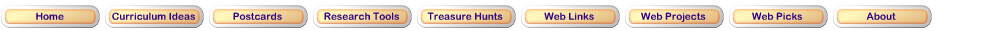Step 1: Introduction to FractionsThis series of Khan Academy videos will illustrate the basics of fractions and how to recognize them.Step 2: Comparing fractionsFractions are a part of your everyday life. They appear in pizzas, candy bars, and even gasoline prices! In this exploration, you will compare fractions with different names.Step 3: What's in-between?Now that you know how to compare the sizes of two fractions, you will explore the space in between two fractions. Are there any fractions bigger than 2/3 but smaller than 3/5? Use this exploration to find out!Step 4: Making things equalIn this final exploration, you will learn about equivalence. How can you tell if two fractions are equal to each other? Many people can name a fraction that is equal to 1/2. Can you name some that are equal to 3/5? What about 9/10?ABCya Test your skill at Equivalent Fractions Bingo.Discovery Education Add the fractions.Illuminations Try your luck at this fraction game.Phet Choose your level and match the fractions.Updated March 14, 2017
© 1996 - 2009 Linda C. Joseph